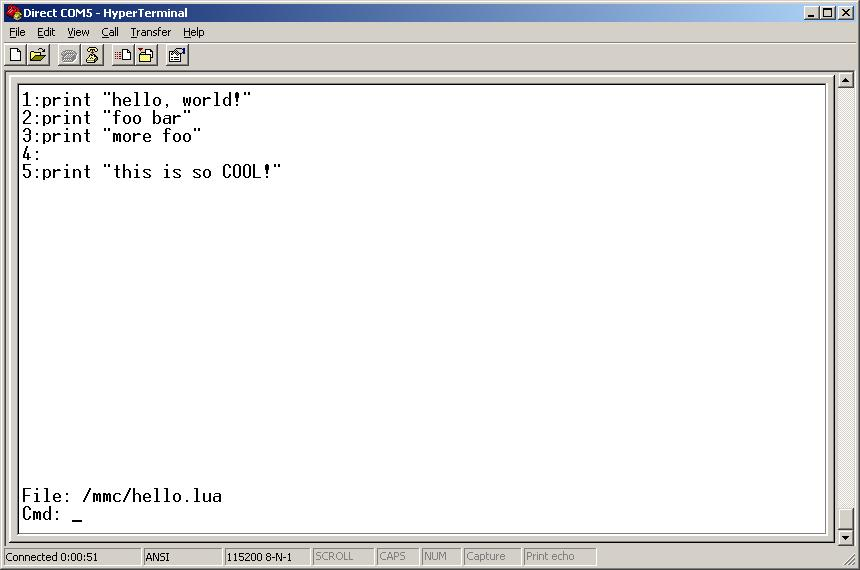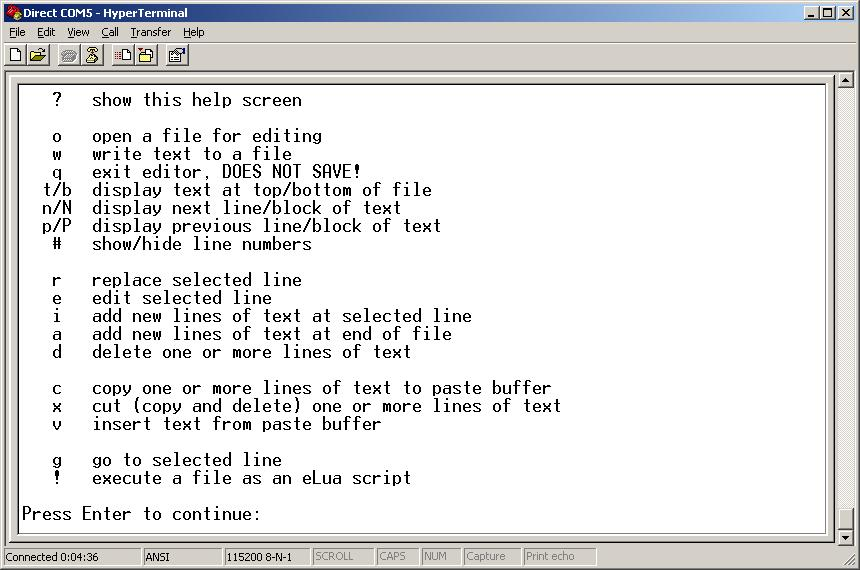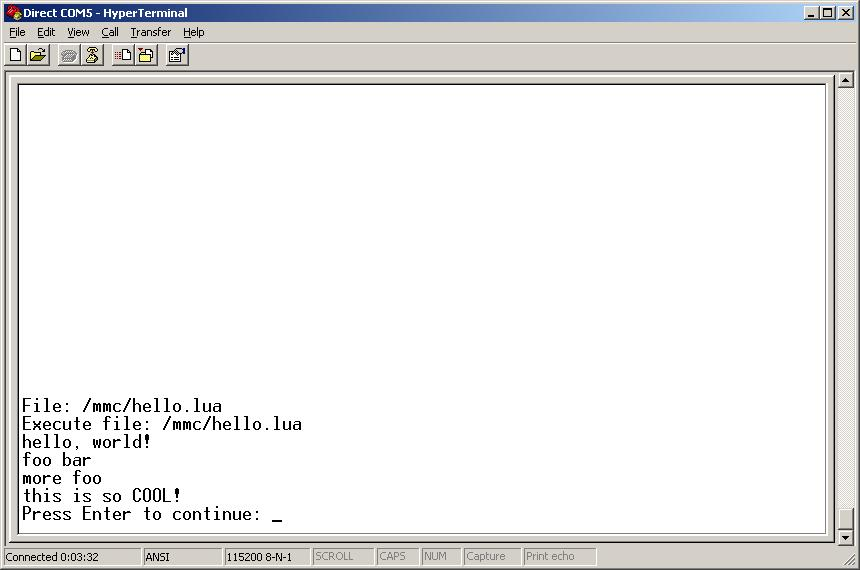A bare-metal editor written in eLua

Update 28 Jul 2013
I've updated the editor code to fix some bugs, notably in the cut/paste operation, and to add some features.

I have started using eLua on some of my embedded ARM boards.  For example, you can find some early mbed testing I did described here.

Early on, I realized that developing on the PC but running on a different target was a pain.  I was constantly shuffling files back and forth, and finding the areas of the source file I wanted to edit meant keeping several windows open.  What I really needed was a text editor that ran on my ARM platform.  And since eLua is such a powerful language, why not write the editor in eLua?  Sounded like a terrific way to learn a bit about eLua.

Please note that I am hardly an expert in eLua.  I've only been using the language for a few weeks, and much of that time was spent with learning the build system and adding hardware to my dev boards.  So I'm not holding this editor up as good eLua coding.  Feel free to send in helpful suggestions to improve the editor.

Features of the editor
The editor is less than 600 lines of eLua code, so it is fairly small.  It is a full-screen text editor that allows you to:
• scroll through the file
• go to a selected line
• delete blocks of text
• insert/append new text into a file
• cut/copy/paste blocks of text within a file
• paste blocks of text into a different file
• edit a selected line of text
• show/hide line numbers
• execute an eLua script (including the file you are editing)

Using the editor
You invoke the editor by launching it as a typical eLua script.  From the eLua shell, enter:
/mmc/edit.lua
(assuming that the editor is on your target board's SD card).  Note that you can also provide the path to a file you want to edit, as in:
/mmc/edit.lua  /mmc/hello.lua

Here is a screenshot showing the editor running in a Hyperterm window:The editor responds instantly to single-character commands.  For example, if I type '?', I immediately see the editor's help screen:Most of these commands are pretty obvious.  I can open a file with the 'o' command, write a file back out with the 'w' command, and quit the editor with the 'q' command.  Note that if you try to exit the editor after you have changed a file, the editor will warn you about unsaved changes and give you a chance to return to the editor.

The commands t, b, n, N, p, and P allow you to move through the text file.  The 'g' command lets you go to a selected line.

There are two ways to modify a selected line.  The 'r' command completely replaces the line; you type in a whole new line of text.  The 'e' command lets you perform substitutions on the selected line.  Substitutions are always of the form: /<old>/<new>/ or \<old>\<new>\.

The '!' command lets you execute an eLua script without leaving the editor.  This is handy if you want to check your current work.  Note that the '!' command executes a file, it cannot execute your text buffer.  If you want to execute the buffer you've been working on but don't want to overwrite the original file, save your work to a temporary file before using the '!' command.  Note that you do not get to supply arguments to the '!' command; you can only enter the name of a file to execute.

Here is a screenshot where I executed the hello.lua script I had been editing:Restrictions and concerns
The editor requires a fair amount of RAM.  Even though it is less than 600 lines long, it will not load on an mbed; not enough memory.  I developed this editor on an eLua binary image for the STM32F4 Discovery board, which has a total of 192 KB of RAM.  This is enough RAM to load the editor and load the editor's source file, so I can edit the editor with the editor.  (Whew!)  I can even execute the editor from within the editor, which is pretty trick, but when I exit the second copy of the editor, I also exit the first copy and return to the eLua shell.

eLua usually uses a serial port as the console, but this editor is very snappy due to eLua's speed.  Screens repaint quickly and navigation through the file is brisk.

Here is the full source for the editor.  Just copy this file to your desktop, start an eLua session on your hardware, then use Xmodem to send this file to your target and save it as edit.lua.

Last updated 28 Jul 2013

lines = {}
pastebuff = {}
showlinenums = true
filepath = ""

CMD_QUIT = string.byte('q')
CMD_OPEN = string.byte('o')
CMD_GOTO = string.byte('g')
CMD_INSERT = string.byte('i')
CHAR_CTRL_Z = 268             -- ctrl-Z
CMD_WRITE = string.byte('w')
CMD_DELETE = string.byte('d')
CMD_TOP = string.byte('t')
CMD_BOTTOM = string.byte('b')
CMD_NEXT_BLK = string.byte('N')
CMD_PREV_BLK = string.byte('P')
CMD_APPEND = string.byte('a')
CMD_HELP = string.byte('?')
CMD_REPLACE = string.byte('r')
CMD_COPY = string.byte('c')
CMD_PASTE = string.byte('v')
CMD_CUT = string.byte('x')
CMD_TOGGLE_NUMS = string.byte('#')
CMD_EDIT = string.byte('e')
CMD_NEXT_LINE = string.byte('n')
CMD_PREV_LINE = string.byte('p')
CMD_EXECUTE = string.byte('!')

CMD_YES = string.byte('y')
CMD_NO = string.byte('n')

local  prevstatusmsg = ""

--LINES_IN_DISPLAY = term.getlines()
LINES_IN_DISPLAY = 22
LINE_ERROR_DISPLAY = LINES_IN_DISPLAY+2
LINE_STATUS = LINES_IN_DISPLAY+1
LINE_CMD = LINES_IN_DISPLAY+2

LINE_ENDING = "\n"

function ShowHelp()
term.clrscr()
print("   " .. string.char(CMD_HELP)     .. "   show this help screen")
print()
print("   " .. string.char(CMD_OPEN)     .. "   open a file for editing")
print("   " .. string.char(CMD_WRITE)    .. "   write text to a file")
print("   " .. string.char(CMD_QUIT)     .. "   exit editor, DOES NOT SAVE!")
print("  " .. string.char(CMD_TOP) .. "/" .. string.char(CMD_BOTTOM)
.. "  display text at top/bottom of file")
print("  " .. string.char(CMD_NEXT_LINE) .. "/" .. string.char(CMD_NEXT_BLK)
.. "  display next line/block of text")
print("  " .. string.char(CMD_PREV_LINE) .. "/" .. string.char(CMD_PREV_BLK)
.. "  display previous line/block of text")
print("   " .. string.char(CMD_TOGGLE_NUMS) .. "   show/hide line numbers")
print()
print("   " .. string.char(CMD_REPLACE)  .. "   replace selected line")
print("   " .. string.char(CMD_EDIT)     .. "   edit selected line")
print("   " .. string.char(CMD_INSERT)   .. "   add new lines of text at selected line")
print("   " .. string.char(CMD_APPEND)   .. "   add new lines of text at end of file")
print("   " .. string.char(CMD_DELETE)   .. "   delete one or more lines of text")
print()
print("   " .. string.char(CMD_COPY)     .. "   copy one or more lines of text to paste buffer")
print("   " .. string.char(CMD_CUT)      .. "   cut (copy and delete) one or more lines of text")
print("   " .. string.char(CMD_PASTE)    .. "   insert text from paste buffer")
print()
print("   " .. string.char(CMD_GOTO)     .. "   go to selected line")
print("   " .. string.char(CMD_EXECUTE)  .. "   execute a file as an eLua script")
print()
term.print(1, LINE_CMD, "Press Enter to continue: ")
repeat
c = term.getchar(term.WAIT)
until (c == term.KC_ENTER)
end

function ModifyLine(linenum, cmd)
if (cmd:sub(1,1) == string.sub('?',1,1)) then
term.clrscr()
print("Enter a substitution string to edit this line.")
print("A substitution string has a pattern and a replacement")
print("string, separated by delimiters.")
print()
print("For example:")
print("  /foo/bar/")
print("will change the first occurence of 'foo' to 'bar'.")
print()
print("You can use either '/' or '\\' as delimiters, but you must")
print("use the same delimiter throughout the string.")
print()
term.print(1, LINE_CMD, "Press Enter to continue: ")
repeat
c = term.getchar(term.WAIT)
until (c == term.KC_ENTER)
else
delim = cmd:sub(1,1)
if ((delim == string.sub('/', 1, 1)) or (delim == string.sub('\\', 1, 1))) then
patend = cmd:find(delim, 2)
if (patend ~= nil) then
pattern = cmd:sub(2, patend-1)
replend = cmd:find(delim, patend+1)
if (replend ~= nil) then
repl = cmd:sub(patend+1, replend-1)
s = lines[linenum]
s = string.gsub(s, pattern, repl, 1)
lines[linenum] = s
filechanged = true
end
end
end
end
end

function ShowErrorAndWait(errmsg)
MoveToLineAndPrint(LINE_ERROR_DISPLAY, errmsg)
repeat
c = term.getchar(term.WAIT)
until (c == term.KC_ENTER)
end

function ShowStatus(statusmsg)
if (statusmsg == nil) then
MoveToLineAndPrint(LINE_STATUS, prevstatusmsg)
else
MoveToLineAndPrint(LINE_STATUS, statusmsg)
prevstatusmsg = statusmsg
end
end

file = io.open(filepath)
if (file == nil) then
ShowErrorAndWait("Cannot open file: " .. filepath)
return
end

repeat
if (line ~= nil) then
table.insert(lines, line)
end
until (line == nil)
file:close()

ShowStatus("File: " .. filepath)
filechanged = false
firstline = 1
end

function SaveFile(savepath)
file = io.open(savepath, "w")
if (file == nil) then
ShowErrorAndWait("Cannot write to file " .. savepath)
return
end

for i, l in ipairs(lines) do
file:write(l, LINE_ENDING)
end
file:flush()
file:close()
end

function DisplayFile(firstline)
term.clrscr()
for i, str in ipairs(lines) do
if (i >= firstline) then
if (showlinenums == true) then
term.print(1, i - firstline + 1, i .. ":" .. str)
else
term.print(1, i - firstline + 1, str)
end
if ((i - firstline + 1) == LINES_IN_DISPLAY) then
break
end
end
end
ShowStatus()
end

function MoveToLineAndPrint(linenum, str)
term.moveto(1, linenum)
term.clreol()
term.print(str)
end

function GetCmd()
repeat
MoveToLineAndPrint(LINE_CMD, "Cmd: ")
c = term.getchar(term.WAIT)

if (c == CMD_QUIT) then
if (filechanged == true) then
MoveToLineAndPrint(LINE_CMD, "File has changed!  Quit without saving? ")
repeat
c = term.getchar(term.WAIT)
until ((c == CMD_YES) or (c == CMD_NO))
end
if ((c == CMD_YES) or (c == CMD_QUIT)) then
done = 1
return
end

elseif (c == CMD_OPEN) then
if (filechanged == true) then
MoveToLineAndPrint(LINE_CMD, "File has changed!  Open without saving? ")
repeat
c = term.getchar(term.WAIT)
until ((c == CMD_YES) or (c == CMD_NO))
end
if ((c == CMD_YES) or (c == CMD_OPEN)) then
MoveToLineAndPrint(LINE_CMD, "File: ")
if ((tfile ~= nil) and (tfile ~= "")) then
lines = {}
filepath = tfile
firstline = 1
end
end
DisplayFile(firstline)

elseif (c == CMD_GOTO) then
MoveToLineAndPrint(LINE_CMD, "Line: ")
if (firstline > #lines - (LINES_IN_DISPLAY+1)) then
firstline = #lines - LINES_IN_DISPLAY + 1
end
if (firstline < 1) then firstline = 1 end
DisplayFile(firstline)

elseif (c == CMD_INSERT) then
if (#lines == 0) then
insertline = 1
else
MoveToLineAndPrint(LINE_CMD, "Insert above which line: ")
end
if (insertline ~= nil) then
firstline = insertline - LINES_IN_DISPLAY/2
if (firstline < 1) then firstline = 1 end
repeat
MoveToLineAndPrint(LINE_CMD, "Text (or ctrl-z): ")
if (text ~= nil) then
table.insert(lines, insertline, text)
insertline = insertline + 1
if ((insertline - firstline) > LINES_IN_DISPLAY) then
firstline = firstline + 1
end
filechanged = true
end
DisplayFile(firstline)
until (text == nil)
end
DisplayFile(firstline)

elseif (c == CMD_WRITE) then
MoveToLineAndPrint(LINE_CMD, "File to write: ")
if (savefile ~= nil) then
SaveFile(savefile)
filechanged = false
filepath = savefile
end
DisplayFile(firstline)

elseif (c == CMD_DELETE) then
if (#lines == 0) then
ShowErrorAndWait("File is empty!")
else
MoveToLineAndPrint(LINE_CMD, "Lines (start stop): ")
if (start ~= nil) then
if (stop == nil) then
stop = start
end
max = #lines
if (start > max) then
ShowErrorAndWait("File only has " .. max .. " lines!")
else
for n = start, stop do
table.remove(lines, start)
filechanged = true
end
end
firstline = start
end
DisplayFile(firstline)
end

elseif (c == CMD_TOP) then
t = firstline
firstline = 1
if (t ~= firstline) then DisplayFile(firstline) end

elseif (c == CMD_BOTTOM) then
t = firstline
firstline = #lines - LINES_IN_DISPLAY + 1
if (firstline < 1) then firstline = 1 end
if (t ~= firstline) then DisplayFile(firstline) end

elseif (c == CMD_NEXT_BLK) then
t = firstline
firstline = firstline + LINES_IN_DISPLAY
if (firstline > #lines) then
firstline = #lines - LINES_IN_DISPLAY + 1
if (firstline < 1) then firstline = 1 end
end
if (t ~= firstline) then DisplayFile(firstline) end

elseif (c == CMD_PREV_BLK) then
t = firstline
firstline = firstline - LINES_IN_DISPLAY
if (firstline < 1) then firstline = 1 end
if (t ~= firstline) then DisplayFile(firstline) end

elseif (c == CMD_NEXT_LINE) then
t = firstline
if (firstline < (#lines - LINES_IN_DISPLAY)) then
firstline = firstline + 1
end
if (t ~= firstline) then DisplayFile(firstline) end

elseif (c == CMD_PREV_LINE) then
t = firstline
if (firstline > 1) then firstline = firstline - 1 end
if (t ~= firstline) then DisplayFile(firstline) end

elseif (c == CMD_APPEND) then
repeat
MoveToLineAndPrint(LINE_CMD, "Text (or ctrl-z): ")
if (text ~= nil) then
table.insert(lines, text)
filechanged = true
end
firstline = #lines - LINES_IN_DISPLAY + 1
if (firstline < 1) then firstline = 1 end
DisplayFile(firstline)
until (text == nil)

elseif (c == CMD_REPLACE) then
MoveToLineAndPrint(LINE_CMD, "Line: ")
currline = tonumber(text)
if ((currline == nil) or (currline < 1)) then
elseif (currline > #lines) then
ShowErrorAndWait("No such line; file only has " .. #lines .. " lines.")
else
firstline = currline - (LINES_IN_DISPLAY /2)
if (firstline < 1) then firstline = 1 end
DisplayFile(firstline)
tmsg = prevstatusmsg
ShowStatus(currline .. ":" .. lines[currline])
MoveToLineAndPrint(LINE_CMD, "Text (or ctrl-z): ")
if (text ~= nil) then
lines[currline] = text
filechanged = true
end
end
DisplayFile(firstline)
ShowStatus(tmsg)

elseif ((c == CMD_COPY) or
(c == CMD_CUT)) then
if (#lines == 0) then
ShowErrorAndWait("File is empty!")
else
MoveToLineAndPrint(LINE_CMD, "Lines (start stop): ")
if (start ~= nil) then
if (stop == nil) then
stop = start
end
max = #lines
if (start > max) then
ShowErrorAndWait("File only has " .. max .. " lines!")
else
pastebuff = {}
for n = start, stop do
table.insert(pastebuff, lines[n])
if (c == CMD_CUT) then table.remove(lines, n) end
end
end
firstline = start
end
DisplayFile(firstline)
end

elseif (c == CMD_INSERT) then
if (#lines == 0) then
insertline = 1
else
MoveToLineAndPrint(LINE_CMD, "Insert above which line: ")
end
if (insertline ~= nil) then
repeat
MoveToLineAndPrint(LINE_CMD, "Text (or ctrl-z): ")
if (text ~= nil) then
table.insert(lines, insertline, text)
insertline = insertline + 1
if (insertline-firstline > 10) then firstline = firstline + 1 end
filechanged = true
end
DisplayFile(firstline)
until (text == nil)
end
DisplayFile(firstline)

elseif (c == CMD_PASTE) then
if (#pastebuff == 0) then
ShowErrorAndWait("No text in paste buffer!")
else
if (#lines == 0) then
insertline = 1
else
MoveToLineAndPrint(LINE_CMD, "Paste above which line: ")
end
if (insertline ~= nil) then
if (insertline > #lines) then
insertline = #lines + 1
end
firstline = insertline - LINES_IN_DISPLAY/2
if firstline < 1 then firstline = 1 end
for _, text in ipairs(pastebuff) do
table.insert(lines, insertline, text)
insertline = insertline + 1
filechanged = true
end
end
end
DisplayFile(firstline)

elseif (c == CMD_TOGGLE_NUMS) then
showlinenums = not showlinenums
DisplayFile(firstline)

elseif (c == CMD_EDIT) then
if (#lines == 0) then
ShowErrorAndWait("No lines in file!")
DisplayFile(firstline)
else
MoveToLineAndPrint(LINE_CMD, "Edit line: ")
if (editline ~= nil) then
if ((editline < 1) or (editline > #lines)) then
ShowErrorAndPrint(LINE_CMD, "File only has " .. #lines .. " lines!")
DisplayFile(firstline)
else
repeat
DisplayFile(firstline)
MoveToLineAndPrint(LINE_CMD, "Subst (" .. editline .. "): ")
if (cmdline ~= nil) then
ModifyLine(editline, cmdline)
end
until (cmdline == nil)
end
end
end

elseif (c == CMD_HELP) then
ShowHelp()
DisplayFile(firstline)

elseif (c == CMD_EXECUTE) then
if (filepath ~= "") then
MoveToLineAndPrint(LINE_CMD, "Execute file (" .. filepath .. "): ")
else
MoveToLineAndPrint(LINE_CMD, "Execute file: ")
end
if (tfile ~= nil) then
if ((tfile == "") and (filepath ~= "")) then
dofile(filepath)
elseif (tfile ~= "") then
dofile(tfile)
end
end
ShowErrorAndWait("Press Enter to continue: ")
DisplayFile(firstline)

else
DisplayFile(1)
end
until (done == 1)
end

done = 0
if (#arg >= 1) then
filepath = arg
end
DisplayFile(1)
GetCmd()
print()

Summary
This is a fun project and a great way to learn about eLua.  I'm sure there are bugs in the above code, so check back now and then for updates, and send me email if you change something; I might add your changes in, as well.

Home